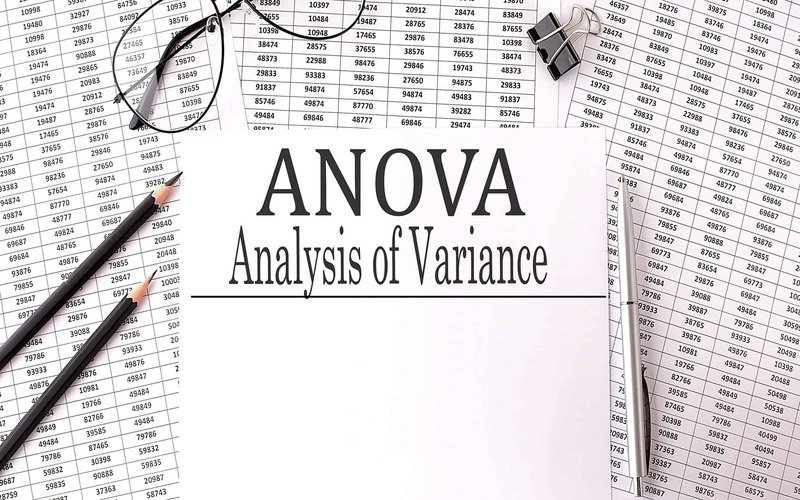Connect with us

Hi, what are you looking for?

DOGE0.070.84%SOL19.370.72%USDC1.000.01%BNB287.900.44%AVAX15.990.06%XLM0.080.37%

# Analysis of Variance (ANOVA) Explanation, Formula, and ApplicationsPhoto: Analysis of Variance (ANOVA) Explanation, Formula, and Applications Photo: Analysis of Variance (ANOVA) Explanation, Formula, and Applications

Analysis of Variance (ANOVA) Explanation, Formula, and Applications

ANOVA is a statistical analysis technique that divides systematic components from random factors to account for the observed aggregate variability within a data set. The presented data set is statistically affected by the systematic factors but not by the random ones. Analysts use the ANOVA test to evaluate the impact of independent factors on the dependent variable in a regression analysis.

Up to 1918, when Ronald Fisher invented the analysis of variance technique, statistical analysis was conducted using the t- and z-test procedures produced in the 20th century.

The t- and z-tests are extended by the analysis of variance (ANOVA), often known as the Fisher analysis of variance. After appearing in Fisher’s book, “Statistical Methods for Research Workers,” the phrase gained widespread recognition in 1925.

It was used in experimental psychology before being applied to more complicated topics.

What is revealed by the analysis of variance?

The ANOVA test is the first stage in examining the variables influencing a certain data set. After the test is complete, an analyst runs additional tests on the procedural elements that demonstrably contribute to the inconsistent nature of the data set. The analyst uses the ANOVA test findings in an F-test to provide more data consistent with the suggested regression models.

Using the ANOVA test, you may compare more than two groups at once to see whether they are correlated. The F statistic, or F-ratio, the outcome of the ANOVA formula, enables the examination of several data sets to ascertain the variability within and across samples.

The outcome of the ANOVA’s F-ratio statistic will be near one if there is no discernible difference between the tested groups, known as the null hypothesis. The F-distribution is the distribution of all potential F statistic values. The numerator degrees of freedom and denominator degrees of freedom are two characteristic numbers describing this distribution function collection.

Example of Using ANOVA

For instance, a researcher may administer tests to students from various universities to determine whether colleges regularly produce better performers than others. To determine whether a product creation technique is more cost-effective than the other in a corporate setting, an R&D researcher may examine two distinct methods.

A variety of variables determines ANOVA test types. It is used when experimental data is required. If statistical software is unavailable, analysis of variance is used, requiring manual computation of ANOVA. It is easy to use and works best with little samples. In many experimental designs, the sample sizes must be identical for all possible factor-level combinations.

When examining three or more variables, an ANOVA is useful. It resembles several two-sample t-tests. However, it produces fewer type I errors and is suitable for various problems. ANOVA comprises dispersing the variation across many sources and groupings differences by comparing the means of each group. It is used with test groups, participants, and between and within groups.

Comparison between One-Way and Two-Way ANOVA

One-way (or unidirectional) and two-way ANOVA are the two primary varieties. ANOVA also comes in several forms. For instance, MANOVA (multivariate ANOVA) differs from ANOVA in that the former evaluates several dependent variables simultaneously while the latter only does so for one. The number of independent variables in your analysis of variance test—one or two—is referred to as one-way or two-way. A one-way ANOVA analyzes how one factor affects just one response variable. It establishes if every sample is the same. The one-way ANOVA is utilized to evaluate any statistically significant differences between the means of three or more independent (unrelated) groups.

The two-way ANOVA expands upon the one-way ANOVA. One independent variable influences one or more dependent variables in a one-way relationship. A two-way ANOVA has two independent variables. A two-way ANOVA, for instance, enables a business to compare employee productivity based on two independent factors: skill level and compensation. It is used to examine the simultaneous effects of two factors and see how the two elements interact.

What distinguishes an ANOVA from a T-test?

ANOVA and T’s tests are different because ANOVA can compare three or more groups, whereas T-tests can only compare two groups at once.

Analysis of Covariance (ANCOVA) – what is it?

Regression and ANOVA are combined in the analysis of covariance. It can be aided by understanding within-group variation that ANOVA tests do not explain.

ANOVA: Does it make any assumptions?

ANOVA tests assume that the data is normally distributed and that each group’s degree of variance is equal. Last but not least, it considers all observations to be produced independently. ANOVA could not help compare groups if these presumptions are incorrect.

ANOVA is useful for comparing more than two groups and discovering connections between them. The method can be applied in academic contexts to evaluate research or finance to forecast future stock value changes. It might be beneficial for sophisticated investors to understand how ANOVA functions and when it could be a valuable tool.

## The Formula for ANOVA is:

F=MST/MSE where: F=ANOVA coefficient MST=Mean sum of squares due to treatment MSE=Mean sum of squares due to error

Conclusion

• A statistical technique called analysis of variance, or ANOVA, divides observed variance data into several components for use in further testing.
• When there are three or more data groups, a one-way ANOVA is used to determine how the dependent and independent variables are related.
• If there is no real difference between the groups, the F-ratio for the ANOVA should be near 1.